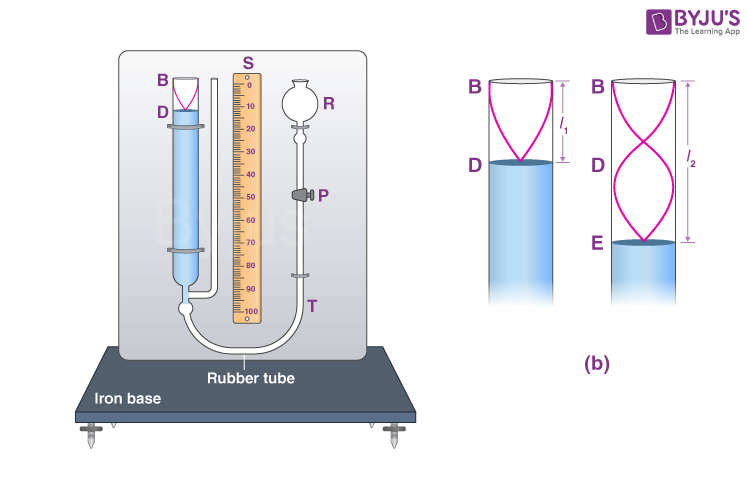# To Find the Speed of Sound in Air at Room Temperature Using a Resonance Tube by Two Resonance Positions

## Aim

To find the speed of sound in air at room temperature using a resonance tube by two resonance positions.

## Apparatus/Materials Required

• Resonance tube
• Thermometer
• Set Squares
• Plumb Line
• Water in a beaker
• Two-timing forks of known frequency

## Diagram## Theory

Let l1 and l2 be the length of the air column for the first and second resonance respectively with a tuning fork of frequency f.

Then,

$$\begin{array}{l}\lambda =2(l_2-l_1)\end{array}$$

The speed is given by the formula

$$\begin{array}{l}v=f\lambda\end{array}$$

Substituting, we get

$$\begin{array}{l}v=2f(l_2-l_1)\end{array}$$

## Procedure

1. By making base horizontal with the help of levelling screws, set the resonance tube vertical.
2. Fix the reservoir R in the uppermost position.
3. Loosen the pinch cock P and fill the reservoir and metallic tube completely with water by a beaker.
4. Tighten the pinch cock, lower the reservoir and fix it in the lowest position.
5. Take a tuning fork of higher frequency

## Observation

The temperature of the air in the air column:

(i) in the beginning ____ °C

(ii) at the end _____°C

The mean temperature is calculated as follows:

$$\begin{array}{l}t=\frac{t_1+t_2}{2}\end{array}$$

Frequency of first tuning fork = f1

Frequency of second tuning fork = f2

 Frequency of tuning fork Resonance No. of observation Position of water level at resonance Mean Length Water level falling (cm) Water level rising (cm) Mean (cm) f1 First 1 2 3 Second 1 2 3 f2 First 1 2 3 Second 1 2 3

## Calculation

From the first tuning fork,

$$\begin{array}{l}v_1=2f_1(l_2′-l_1′)\end{array}$$

From the second tuning fork,

$$\begin{array}{l}v_2=2f_2(l_2″-l_1″)\end{array}$$

The mean velocity at room temperature is given as follows:

$$\begin{array}{l}v=\frac{v_1+v_2}{2}\end{array}$$

## Result

At room temperature, the velocity of sound in air is _____ m/s.

## Viva Voce

1. What is the working principle of the resonance tube?

It works on the principle of resonance of the air column with a tuning fork.

2. What types of waves are produced in the air column?

Longitudinal stationary waves are produced in the air column.

3. Do you find the velocity of sound in air column or in the water column?

The velocity of sound is found in the air column above the water column.

4. What are the possible errors in the result?

The two possible errors in the result are:

(i) The enclosed air in the air column is denser than the outside air, this may reduce the velocity of air.

(ii) The humidity above the enclosed water column may increase the velocity of sound.

5. Will the result be affected if we take other liquids than water?